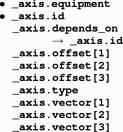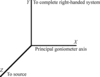International
Tables for
Crystallography
Volume G
Definition and exchange of crystallographic data
Edited by S. R. Hall and B. McMahon

International Tables for Crystallography (2006). Vol. G. ch. 3.7, pp. 201-202

## Section 3.7.3. Axes

H. J. Bernsteina*

aDepartment of Mathematics and Computer Science, Kramer Science Center, Dowling College, Idle Hour Blvd, Oakdale, NY 11769, USA
Correspondence e-mail: yaya@bernstein-plus-sons.com

### 3.7.3. Axes

| top | pdf |

The category describing the axes required to specify the data collection is as follows:

 AXIS group AXIS

Data items in this category are as follows:

AXISThe bullet () indicates a category key. The arrow () is a reference to a parent data item.

Data items in the AXIS category record the information required to describe the goniometer, detector, source and other axes needed to specify a data collection. The location of each axis is specified by two vectors: the axis itself, given as a unit vector, and an offset to the base of the unit vector. These vectors are referenced to a right-handed laboratory coordinate system with its origin in the specimen, as shown in Fig. 3.7.3.1.Figure 3.7.3.1 | top | pdf |AXIS laboratory coordinate system. The origin is centred in the specimen.

The X axis is aligned to the mechanical axis pointing from the specimen along the principal axis of the goniometer.

The Z axis is defined next. The Z axis is derived from the source axis (the axis running from the sample to the source). If the source axis is orthogonal to the X axis, the source axis is the Z axis. If the source axis is not orthogonal to the X axis, the Z axis is the component of the source axis orthogonal to the X axis. The direction is chosen to form an acute angle with the source axis.

The Y axis is defined last. The Y axis completes an orthogonal right-handed system defined by the X axis and the Z axis (see below).

These axes are based on the goniometer, not on the orientation of the detector, gravity etc. The vectors necessary to specify all other axes are given by sets of three components in the order (X, Y, Z). If the axis involved is a rotation axis, it is right-handed, i.e. as one views the object to be rotated from the origin (the tail) of the unit vector, the rotation is clockwise. If a translation axis is specified, the direction of the unit vector specifies the sense of positive translation.

Note: This choice of coordinate system is similar to but significantly different from the choice in MOSFLM (Lesley & Powell, 2003), in which X is along the X-ray beam (our Z axis) and Z is along the rotation axis.

All rotations are given in degrees and all translations are given in millimetres.

Axes may be dependent on one another. The X axis is the only axis that is strictly connected to the hardware. All other axes are specified by the positions they would assume when the axes upon which they depend are at their zero points.

When specifying detector axes, each axis is specified relative to the beam centre. The location of the beam centre on the detector should be given in the DIFFRN_DETECTOR category in millimetres from the (0, 0) corner of the detector and should be corrected for distortion.

It should be noted that many different origins arise in the definition of an experiment. In particular, as noted above, we need to specify the location of the beam centre on the detector in terms of the origin of the detector, which is, of course, not coincident with the centre of the sample.

Each axis is uniquely identified by the values of _axis.id and of _axis.equipment. An axis may be a translation axis, a rotation axis or an axis for which the mode of motion is not relevant. The type of axis is specified by the value of _axis.type. The base of the axis is specified by the point in the laboratory coordinate system given by the values of _axis.offset, _axis.offset and _axis.offset, and the direction of the axis from that base, as a dimensionless unit vector, is given by _axis.vector, _axis.vector and _axis.vector.

### ReferencesLeslie, A. G. W. & Powell, H. (2003). MOSFLM V6.11. http://www.ccp4.ac.uk/dist/x-windows/Mosflm .Google Scholar